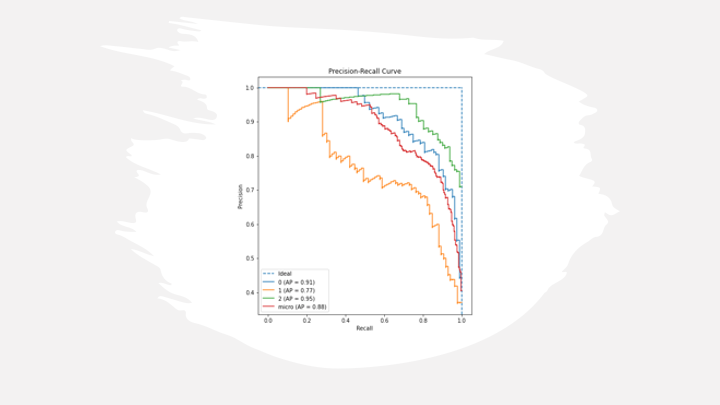Join thousands of AI enthusiasts and experts at the Learn AI Community.

## PublicationLatest

# Precision-Recall Curve

Last Updated on January 6, 2023 by Editorial Team

Last Updated on October 3, 2022 by Editorial Team

#### Author(s): Saurabh Saxena

Originally published on Towards AI the World’s Leading AI and Technology News and Media Company. If you are building an AI-related product or service, we invite you to consider becoming an AI sponsor. At Towards AI, we help scale AI and technology startups. Let us help you unleash your technology to the masses.

#### PR Curve, AUC-PR, and AP

Evaluation of any model is vital. When it comes to classification models, be they binary or multi-class, we have a wide range of metrics available at our disposal. If we have a balanced dataset, you might choose Accuracy. If True Prediction is more important, precision, recall, specificity, or F1 will be the choice. All the metrics mentioned here use prediction class for evaluation and not prediction score. However, some metrics use prediction scores like Precision-Recall Curve and ROC.

#### Precision-Recall Curve:

The precision-recall curve shows the tradeoff between precision and recalls for different thresholds. It is often used in situations where classes are heavily imbalanced. For example, if an observation is predicted to belong to the positive class at probability > 0.5, it is labeled as positive. However, we could choose any probability threshold between 0 and 1. A precision-recall curve helps to visualize how threshold affects classifier performance.

To understand precision and recall, let’s quickly refresh our memory on the possible outcomes in a binary classification problem by referring to the Confusion Matrix.

Precision is referred to the proportion of correct predictions among all predictions for a particular class.

The recall is referred to the proportion of examples of a particular class that has been predicted by the model as belonging to that class.

A model with high precision and recall will return very few results, but most of the predictions are correct.

However, a model with low precision and high recall return many results, but most of the predictions will be incorrect. An Ideal model will have high precision and high recall and will return many results with all correctly predicted, while a baseline model will have very low precision.

`from sklearn.datasets import make_classificationfrom sklearn.model_selection import train_test_splitfrom sklearn.linear_model import LogisticRegressionfrom sklearn.metrics import precision_recall_curvefrom sklearn.metrics import PrecisionRecallDisplay`
`X, y = make_classification(n_samples=1000, n_classes=2,                           random_state=1)X_train, X_test, y_train, y_test = train_test_split(X, y,                                                    test_size=.2,                                                    random_state=2)lr = LogisticRegression()lr.fit(X_train, y_train)y_pred = lr.predict(X_test)y_pred_prob = lr.predict_proba(X_test)y_pred_prob = y_pred_prob[:,1]`
`precision, recall, threshold = precision_recall_curve(y_test,                                                       y_pred_prob)prd = PrecisionRecallDisplay(precision, recall)prd.plot()`

AP and AUC-PR

Average Precision summarizes the PR curve into a single metric as the weighted mean of the precision achieved at each threshold value.

where Pn and Rn are the precision and recall at the n^th threshold.

AUC-PR stands for Area Under the Curve-Precision Recall, and it is the trapezoidal area under the plot. AP and AUC-PR are similar ways to summarize the PR curve into a single metric.

A high AP or AUC represents the high precision and high recall for different thresholds. The value of AP/AUC fluctuates between 1 (ideal model) and 0 (worst model).

`from sklearn.metrics import average_precision_scoreaverage_precision_score(y_test, y_pred_prob)`
`Output:0.927247516623891`

We can combine the PR score with the graph.

`ap = average_precision_score(y_test, y_pred_prob)prd = PrecisionRecallDisplay(precision, recall, average_precision=ap)prd.plot()`

Precision-Recall curves typically use two classes for evaluation, and for multi-class or multi-label classification line curve will be drawn per class, and AP or AUC for each class will be helpful for ranking among the classes. However, to summarize multi-class in one metric, micro, macro, and weighted Precision-Curve and AP/AUC can be calculated. Please refer to Multi-class Model Evaluation with Confusion Matrix and Classification Report to understand micro, macro, and weighted metrics.

Below is the python code to create and plot PR for multi-class classification.

`from sklearn.datasets import make_classificationfrom sklearn.preprocessing import label_binarizefrom sklearn.model_selection import train_test_splitfrom sklearn.linear_model import LogisticRegressionfrom sklearn.multiclass import OneVsRestClassifier`
`# Load DatasetX, y = make_classification(n_samples=1000, n_classes=2,                           random_state=1)y = label_binarize(y, classes=[0,1,2])X_train, X_test, y_train, y_test = train_test_split(X, y,                                                    test_size=.2,                                                    random_state=2)lr = LogisticRegression()ovr = OneVsRestClassifier(lr)ovr.fit(X_train, y_train)y_pred = ovr.predict(X_test)y_pred_prob = ovr.predict_proba(X_test)`
`precision, recall, threshold, ap, labels = pr_curve(y_test,                                                    y_pred_prob,                                                     labels=[0,1,2])pr_curve_plot(precision, recall, threshold, ap, labels)`

References:Precision-Recall Curve was originally published in Towards AI on Medium, where people are continuing the conversation by highlighting and responding to this story.

Join thousands of data leaders on the AI newsletter. It’s free, we don’t spam, and we never share your email address. Keep up to date with the latest work in AI. From research to projects and ideas. If you are building an AI startup, an AI-related product, or a service, we invite you to consider becoming a sponsor.

Published via Towards AI